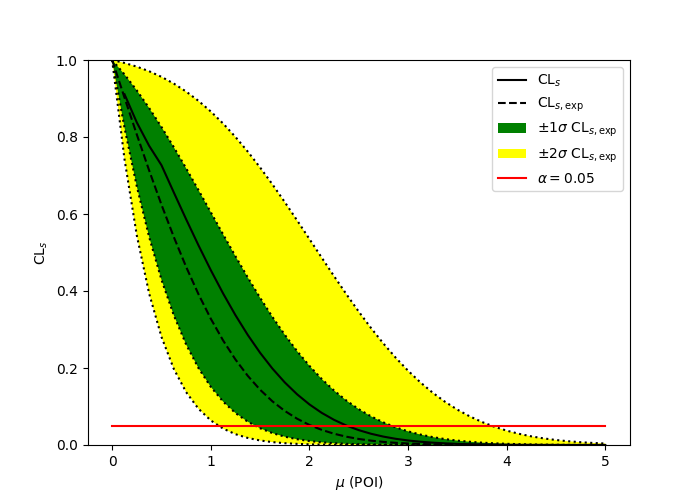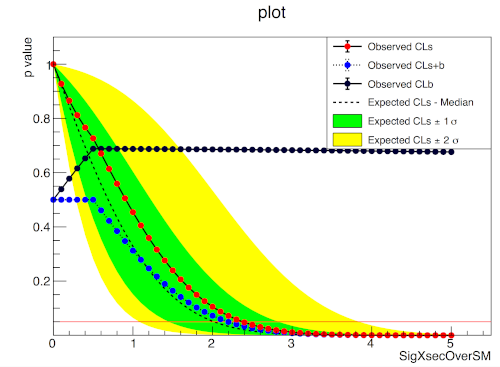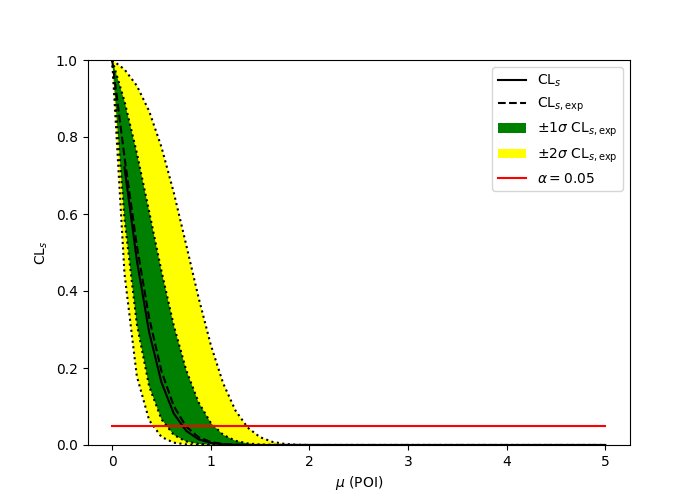Fork me on GitHub# pure-python fitting/limit-setting/interval estimation HistFactory-style¶

The HistFactory p.d.f. template [CERN-OPEN-2012-016] is per-se independent of its implementation in ROOT and sometimes, it’s useful to be able to run statistical analysis outside of ROOT, RooFit, RooStats framework.

This repo is a pure-python implementation of that statistical model for multi-bin histogram-based analysis and its interval estimation is based on the asymptotic formulas of “Asymptotic formulae for likelihood-based tests of new physics” [arXiv:1007.1727]. The aim is also to support modern computational graph libraries such as PyTorch and TensorFlow in order to make use of features such as autodifferentiation and GPU acceleration.

## Hello World¶

This is how you use the pyhf Python API to build a statistical model and run basic inference:

>>> import pyhf
>>> model = pyhf.simplemodels.hepdata_like(signal_data=[12.0, 11.0], bkg_data=[50.0, 52.0], bkg_uncerts=[3.0, 7.0])
>>> data = [51, 48] + model.config.auxdata
>>> test_mu = 1.0
>>> CLs_obs, CLs_exp = pyhf.infer.hypotest(test_mu, data, model, qtilde=True, return_expected=True)
>>> print(f"Observed: {CLs_obs}, Expected: {CLs_exp}")
Observed: 0.05251497423736956, Expected: 0.06445320535890459


Alternatively the statistical model and observational data can be read from its serialized JSON representation (see next section).

>>> import pyhf
>>> import requests
>>> wspace = pyhf.Workspace(requests.get('https://git.io/JJYDE').json())
>>> model = wspace.model()
>>> data = wspace.data(model)
>>> test_mu = 1.0
>>> CLs_obs, CLs_exp = pyhf.infer.hypotest(test_mu, data, model, qtilde=True, return_expected=True)
>>> print(f"Observed: {CLs_obs}, Expected: {CLs_exp}")
Observed: 0.3599840922126626, Expected: 0.3599840922126626


Finally, you can also use the command line interface that pyhf provides which should produce the following JSON output:

$cat << EOF | tee likelihood.json | pyhf cls { "channels": [ { "name": "singlechannel", "samples": [ { "name": "signal", "data": [12.0, 11.0], "modifiers": [ { "name": "mu", "type": "normfactor", "data": null} ] }, { "name": "background", "data": [50.0, 52.0], "modifiers": [ {"name": "uncorr_bkguncrt", "type": "shapesys", "data": [3.0, 7.0]} ] } ] } ], "observations": [ { "name": "singlechannel", "data": [51.0, 48.0] } ], "measurements": [ { "name": "Measurement", "config": {"poi": "mu", "parameters": []} } ], "version": "1.0.0" } EOF { "CLs_exp": [ 0.0026062609501074576, 0.01382005356161206, 0.06445320535890459, 0.23525643861460702, 0.573036205919389 ], "CLs_obs": 0.05251497423736956 }  ## What does it support¶ Implemented variations: • ☑ HistoSys • ☑ OverallSys • ☑ ShapeSys • ☑ NormFactor • ☑ Multiple Channels • ☑ Import from XML + ROOT via uproot • ☑ ShapeFactor • ☑ StatError • ☑ Lumi Uncertainty Computational Backends: • ☑ NumPy • ☑ PyTorch • ☑ TensorFlow • ☑ JAX Optimizers: • ☑ SciPy (scipy.optimize) • ☑ MINUIT (iminuit) All backends can be used in combination with all optimizers. Custom user backends and optimizers can be used as well. ## Todo¶ • ☐ StatConfig • ☐ Non-asymptotic calculators results obtained from this package are validated against output computed from HistFactory workspaces ## A one bin example¶ import pyhf import numpy as np import matplotlib.pyplot as plt import pyhf.contrib.viz.brazil pyhf.set_backend("numpy") model = pyhf.simplemodels.hepdata_like( signal_data=[10.0], bkg_data=[50.0], bkg_uncerts=[7.0] ) data = [55.0] + model.config.auxdata poi_vals = np.linspace(0, 5, 41) results = [ pyhf.infer.hypotest(test_poi, data, model, qtilde=True, return_expected_set=True) for test_poi in poi_vals ] fig, ax = plt.subplots() fig.set_size_inches(7, 5) ax.set_xlabel(r"$\mu$(POI)") ax.set_ylabel(r"$\mathrm{CL}_{s}$") pyhf.contrib.viz.brazil.plot_results(ax, poi_vals, results)  pyhfROOT## A two bin example¶ import pyhf import numpy as np import matplotlib.pyplot as plt import pyhf.contrib.viz.brazil pyhf.set_backend("numpy") model = pyhf.simplemodels.hepdata_like( signal_data=[30.0, 45.0], bkg_data=[100.0, 150.0], bkg_uncerts=[15.0, 20.0] ) data = [100.0, 145.0] + model.config.auxdata poi_vals = np.linspace(0, 5, 41) results = [ pyhf.infer.hypotest(test_poi, data, model, qtilde=True, return_expected_set=True) for test_poi in poi_vals ] fig, ax = plt.subplots() fig.set_size_inches(7, 5) ax.set_xlabel(r"$\mu$(POI)") ax.set_ylabel(r"$\mathrm{CL}_{s}\$")
pyhf.contrib.viz.brazil.plot_results(ax, poi_vals, results)


pyhfROOT## Installation¶

To install pyhf from PyPI with the NumPy backend run

python -m pip install pyhf


and to install pyhf with all additional backends run

python -m pip install pyhf[backends]


or a subset of the options.

To uninstall run

python -m pip uninstall pyhf


## Questions¶

If you have a question about the use of pyhf not covered in the documentation, please ask a question on Stack Overflow with the [pyhf] tag, which the pyhf dev team watches.If you believe you have found a bug in pyhf, please report it in the GitHub Issues. If you’re interested in getting updates from the pyhf dev team and release announcements you can join the pyhf-announcements mailing list.

## Citation¶

As noted in Use and Citations, the preferred BibTeX entry for citation of pyhf is

@software{pyhf,
author = "{Heinrich, Lukas and Feickert, Matthew and Stark, Giordon}",
title = "{pyhf: v0.5.3}",
version = {0.5.3},
doi = {10.5281/zenodo.1169739},
url = {https://github.com/scikit-hep/pyhf},
}


## Authors¶

pyhf is openly developed by Lukas Heinrich, Matthew Feickert, and Giordon Stark.

Please check the contribution statistics for a list of contributors.

## Milestones¶

• 2020-07-28: 1000 GitHub issues and pull requests. (See PR #1000)

## Acknowledgements¶

Matthew Feickert has received support to work on pyhf provided by NSF cooperative agreement OAC-1836650 (IRIS-HEP) and grant OAC-1450377 (DIANA/HEP).